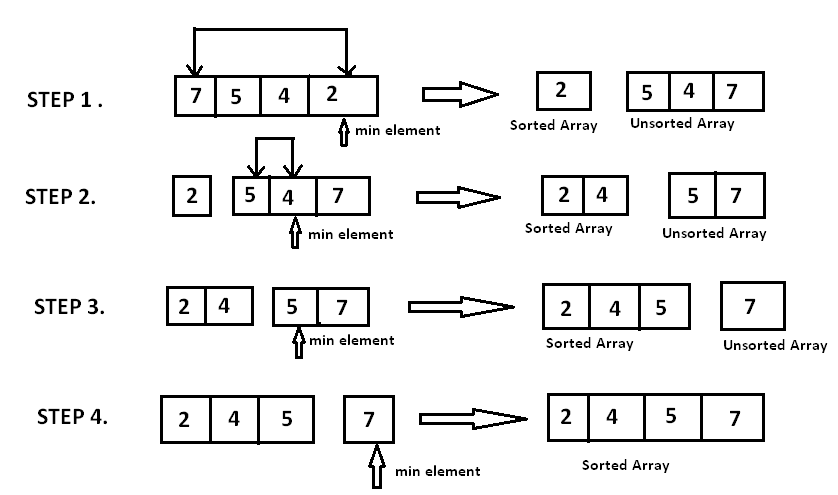# Sorting Algorithm - Selection sort Implementation

Today I am going to show the way to implement the Selection Sort algorithm.

## Selection sort

The algorithm will divide the array into a sorted and unsorted partition. By traverse, the array looks for the largest element in the unsorted partition, and then swap it with the last element in the unsorted partition. Swapped elements will be its correct sorted partition.## Implementation

### Ascending

• Init the `lastUnsortedIndex` (last index of an unsorted partition)

• Init the `largestUnsortedIndex` (index of the largest element in the unsorted partition). The initial value equals 0.

• In a descending loop from the `lastUnsortedIndex` til 0, we set the initial value of `largestUnsortedIndex` is 0, then we start another ascending loop from 1 to lastUnsortedIndex.

Then we compare the value of current index vs the value of `largestUnsortedIndex`.

• If the value of current index greater than the value of `largestUnsortedIndex`, then the `largestUnsortedIndex = current index`

When the ascending loop ends, we swap the value of `largestUnsortedIndex` with the value of `lastUnsortedIndex`.

• The algorithm ends when `lastUnsortedIndex = 0`.

### Descending

• Init the `firstUnsortedIndex` (first index of unsorted partition - means 0)

• Init the `largestUnsortedIndex` (index of the largest element in the unsorted partition).

• In a ascending loop from the `firstUnsortedIndex` to length of array, we set the initial value of `largestUnsortedIndex` equals `firstUnsortedIndex`, then we start another ascending loop from next element of `firstUnsortedIndex` (firstUnsortedIndex + 1) to length of array.

Then we compare the value of current index vs the value of `largestUnsortedIndex`.

• If the value of current index greater than the value of `largestUnsortedIndex`, then the `largestUnsortedIndex = current index`

When the ascending loop ends, we swap the value of `largestUnsortedIndex` with the value of `firstUnsortedIndex`.

• The algorithm ends when `firstUnsortedIndex = length of array`.